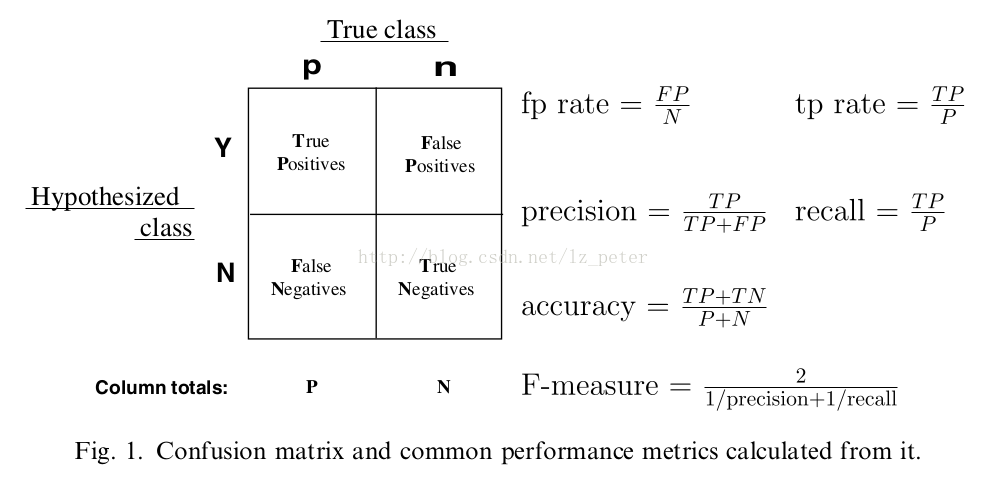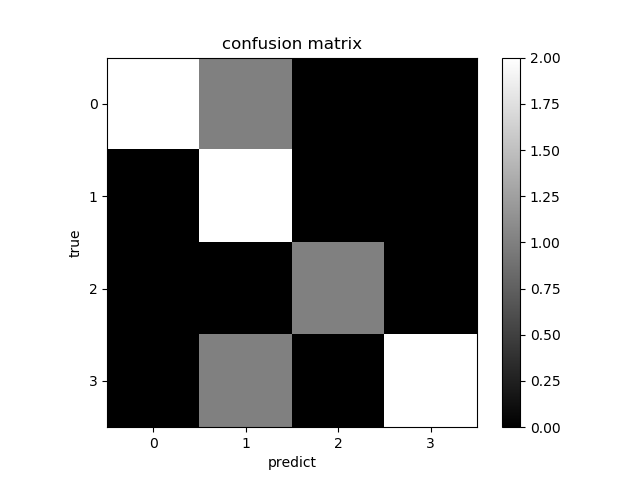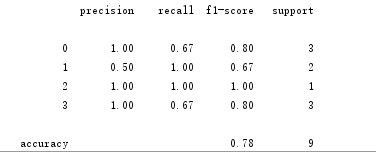# 对模型评估中的准确率、精准率和召回率描述与理解Python 同时被 2 个专栏收录50 篇文章 1 订阅• TP：样本为正，预测结果为正；
• FP：样本为负，预测结果为正；
• TN：样本为负，预测结果为负；
• FN：样本为正，预测结果为负。

### 补充：

import numpy as np
import pandas as pd
import matplotlib.pyplot as plt

np.random.seed(37)

y_true = [1, 0, 0, 2, 1, 0, 3, 3, 3]
y_pred = [1, 1, 0, 2, 1 ,0, 1, 3, 3]

from sklearn.metrics import confusion_matrix
confusion_mat = confusion_matrix(y_true, y_pred)
print(confusion_mat)

def plot_confusion_matrix(confusion_mat):
plt.imshow(confusion_mat, interpolation='nearest', cmap = plt.cm.gray)
plt.title('confusion matrix')
plt.colorbar()
tick_marks = np.arange(confusion_mat.shape)
plt.xticks(tick_marks, tick_marks)
plt.yticks(tick_marks, tick_marks)
plt.ylabel('true')
plt.xlabel('predict')
plt.show()

plot_confusion_matrix(confusion_mat)from sklearn.metrics import classification_report
target_names = ['0', '1', '2', '3']
print(classification_report(y_true, y_pred, target_names=target_names))02-052424
06-1484009-062827
08-222076
02-271万+
06-10215
11-262126
10-1426
11-224036
09-295万+
08-291605
03-182390
07-122501
10-081万+
04-121万+
09-163442
05-201124
07-2994点击重新获取扫码支付余额充值